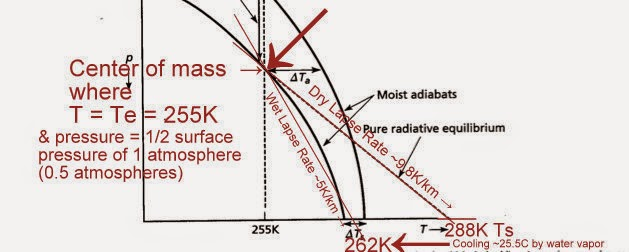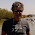Monday, December 1, 2014

Why increased water vapor decreases the lapse rate by half to cause surface cooling of up to 25.5C

First a figure from a paper describing the conventional radiative greenhouse theory:The conventional radiative GHE explanation, which even in the conventional explanation shows the ~5K/km wet adiabatic lapse rate compared to the ~9.8K/km dry adiabatic lapse rate causes surface cooling. The flaw in this conventional theory is that the ERL is set by greenhouse gases rather than the center of mass of the atmosphere.
Now that same figure annotated to show why the ERL height is set at the center of mass of the atmosphere by gravity/pressure, not greenhouse gases, and why the wet adiabatic lapse rate from the ERL to the surface causes surface cooling:Above figure corrected to show what the greenhouse equation and mass/gravity/pressure theory of the GHE predict, and which exactly reproduces the US Standard Atmosphere Database to within 0.02K at every single meter all the way from the surface to the ERL at ~5100 meters and then up to the top of the troposphere at ~11,000-12,000 meters (on average).The ERL height is set by the height where all of the following converge: T= Te = 255K and center of mass where P = Ps/2 = 0.5 atmospheres, and the average lapse rate [=-g/Cp] in that location, and is not dependent upon greenhouse gas radiative forcing.

1.MS,

Do you agree that in so far as the latent heat of evaporation increases uplift beyond that which would have occurred in dry air then that latent heat gets converted to PE (more work done against gravity) which is then recovered as KE in dry air on the descent ?

It would follow that the net cooling effect of water vapour for the system as a whole (as opposed to the surface from which evaporation occurred) is limited to radiation to space from the vapour and the condensate but that that is not the whole story due to the unradiated portion being returned on the descent.

For the system as a whole there must be an ideal lapse rate determined solely by mass and gravity and I am wondering whether our watery planet, in distorting the lapse rate one way in ascent is maybe also distorting the dry lapse rate on the descent such that both actual lapse rates diverge from the ideal lapse rate to an equal and opposite extent.

1.That's a good way of explaining it.

I propose a conceptual way of looking at it as the average LR 6.5 is in-between the wet LR 5 of ascending wet packets of air which dry out on the way up, while accumulating gravitational potential energy in the process, and then these dry packets descend using that gravitational PE along a dry adiabatic LR, so on average the average lapse rate would be intermediate between the wet and dry rates.

2.The only this could work is if Miskolczi is right, and the net greenhouse effect on earth remains constant with time. Any slight increase from CO2 is then quickly offset by H2O.

Not impossible but as yet unproven.

1.It would also work in a 100% N2 atmosphere:

With a 100% N2 atmosphere, the surface temperature would be Ts = Te + (the gravito-thermal GHE of 33K) = at least 288K (and I calculate around 306K due to the lack of WV decreasing the lapse rate to the average on Earth)

And that 288K+ at the surface would then radiate directly from the surface to space due to the absence of GHGs.

A perfect example of how the radiative GHE has things backwards, GHGs cool the planet by increasing radiative surface area and Cp.

2.I think you mean that in the absence of GHGs the surface at 288K would recycle 33K in and out of adiabatic convective overturning and radiate 255K to space.

If water vapour distorts the average lapse rate in ascent and an equal and opposite amount on the descent then the net effect would be zero would it not ?

So you don't need to propose 306 K without water vapour.

In so far as water vapour and its condensate does work as a GHG then it does potentially cool the system but convective changes negate that to leave mass in absolute control.

And if mass is the primary cause of the greenhouse effect then we would never notice any effect from GHGs anyway.

GHGs do have some effect from having different specific gravities to O2 and N2 but then that is a mass related issue and not a radiative one.

3.Clive, what do you mean with "unproven"? I thought that Miskolczi had based his theory on observational data and that therefore it is his theory that needs to be falsified. I haven't followed that debate in a long time, do you have some updates?

4.I think some climate scientist was dragged out to find an 'error' in Miskolczi complex mathematical derivation. I rather liked his simple premise that the normalised greenhouse effect on earth is 1/3 and will always remain so while oceans cover 70% of the surface.

5.Thanks Clive for the information, I think I found what you were referring to: